# Polygons and its Applications

This set of MCQs helps you brush up on important math topics and prepare you to dive into skill practice.

Start Quiz

A ________ is any polygon that has 4 sides.

Hexagon

Parallelogram

Trapezoid

The figure is a parallelogram.
Solve for x.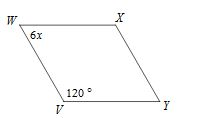8

10

5

4

If the figure is a rectangle, the value of x is.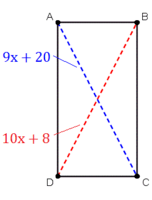14

13

12

28

Solve for the variables.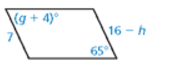g=25, h=9

g=61, h=25

g=61, h=9

g=9, h=61

Solve for x in the rectangle.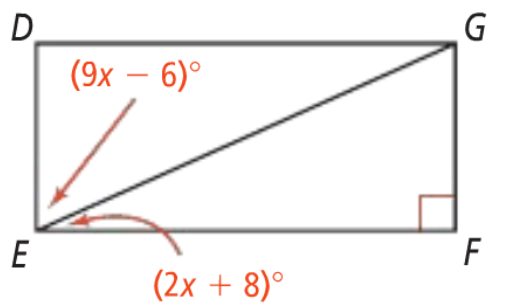10

7

3

8

In parallelogram ABCD, CD is smaller than BC as shown below: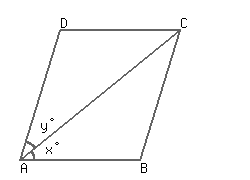x > y

y > x

y = x

y = x = 0

In a parallelogram ABCD, the bisector of ∠A also bisects the side BC. If AB = 9 cm, find the length of side AD.

36

18

15

30

The perimeter of a parallelogram is 120 cm. If one side exceeds the other side by 8 cm, what are the sides of the parallelogram?

26 cm, 34 cm

26 cm, 24 cm

30 cm, 48 cm

36 cm, 34 cm

Quiz/Test Summary
Title: Polygons and its Applications
Questions: 8
Contributed by: